# RD Sharma Solutions - Chapter 13 - Proft, Loss, Discount and Value Added Tax (VAT) (Part -2), Class8 Notes | Study RD Sharma Solutions for Class 8 Mathematics - Class 8

## Class 8: RD Sharma Solutions - Chapter 13 - Proft, Loss, Discount and Value Added Tax (VAT) (Part -2), Class8 Notes | Study RD Sharma Solutions for Class 8 Mathematics - Class 8

The document RD Sharma Solutions - Chapter 13 - Proft, Loss, Discount and Value Added Tax (VAT) (Part -2), Class8 Notes | Study RD Sharma Solutions for Class 8 Mathematics - Class 8 is a part of the Class 8 Course RD Sharma Solutions for Class 8 Mathematics.
All you need of Class 8 at this link: Class 8

PAGE NO 13.26:

Question 1:

Find the S.P. if
(i) M.P. = Rs 1300 and Discount = 10%
(ii) M.P. = Rs 500 and Discount = 15%

(i) We know that SP = MP−Discount

Discount% = Discount/MP × 100

Discount =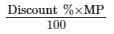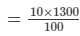So, SP = Rs.(1300−(10/100 × 1300))

= 1300−130 = Rs. 1170

(ii) We know that SP = MP− Discount

Discount % = Discount/MP × 100

Discount =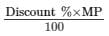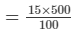So, SP = Rs.( 500−(15/100 × 500)) = Rs.(500−75)

= Rs. 425

PAGE NO 13.26:

Question 2:

Find the M.P. if
(i) S.P. = Rs 1222 and Discount = 6%
(ii) S.P. = Rs 495 and Discount = 1%

(i) Given, SP = Rs 1222

Discount = 6%

So, MP =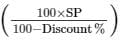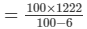= Rs. 1300

(ii) Given,SP = Rs. 495

Discount = 1%

So, MP =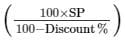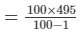= Rs. 500

PAGE NO 13.26:

Question 3:

Find discount in percent when
(i) M.P. = Rs 900 and S.P. = Rs 873
(ii) M.P. = Rs 500 and S.P. = Rs 425

(i) We know that SP = MP−Discount

So, 873 = 900−Discount

Therefore, Discount = Rs. (900−873)

= Rs. 27

Discount% = Discount/MP × 100%

= 27/900 × 100%

= 3%

(ii) We know that SP = MP−Discount

So, 425 = 500−Discount

Therefore, Discount = Rs.(500−425)

= Rs. 75

Discount% = Discount/MP × 100%

= 75/500 × 100%

= 15%

PAGE NO 13.26:

Question 4:

A shop selling sewing machines offers 3% discount on all cash purchases. What cash amount does a customer pay for a sewing machine the price of which is marked as Rs 650.

Discount  =  3%

Marked price = Rs. 650

Now, 3% of the MP  =  3/100 × 650

= Rs 19.50

So, SP = MP−Discount = 650−19.50

= Rs 630.50

PAGE NO 13.26:

Question 5:

The marked price of a ceiling fan is Rs 720. During off season, it is sold for Rs 684. Determine the discount percent.

Given, MP of the ceiling fan = Rs. 720 SP of the ceiling fan =  Rs. 684

Since SP = MP−Discount,

Discount = MP−SP = Rs. (720−684)

= Rs. 36

Discount% = (Discount/MP) × 100%

= 36/720 × 100%

= 5%

PAGE NO 13.26:

Question 6:

On the eve of Gandhi Jayanti a saree is sold for Rs 720 after allowing 20% discount. What is its marked price?

Given,SP of the saree  = Rs. 720

Discount on the saree = 20%

We know, Discount% = Discount/MP × 100

Or, Discount =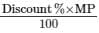Let the MP of the saree be Rs.  x

Therefore, Discount = 20/100 x = Rs. 0.02 x

Since S.P = MP− Discount,

720 = x −0.20 x 720 = 0.80 x

x = 720/0.80

= Rs. 900

Thus, the MP of the saree is Rs. 900.

PAGE NO 13.26:

Question 7:

After allowing a discount of 7.5% on the marked price, an article is sold for Rs 555. Find its markd price.

Given,SP of the article = Rs. 555

Discount = 7.5%

Let the MP of the article be Rs.  x .

Therefore, Discount =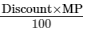= Rs.7.5x/100

= Rs. 0.075 x

Since SP = MP− Discount,

555 = x −0.075 x

555 = 0.925 x

x = 555/0.925

= Rs. 600

Thus, the MP of the article is Rs. 600.

PAGE NO 13.26:

Question 8:

A shopkeeper allows his customers 10% off on the marked price of goods and still gets a profit of 25%. What is the actual cost to him of an article marked Rs 250?

Let the CP of the article be Rs.  x .

MP of the article  =  Rs. 250

Discount = 10%

Discount =  10% of 250 = 0.10 × 250 = Rs. 25

SP = MP−Discount = 250−25 = Rs. 225

Given,Profit = 25%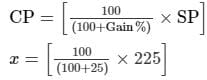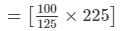= Rs. 180

Thus, the CP of the article is Rs. 180.

PAGE NO 13.26:

Question 9:

A shopkeeper allows 20% off on the marked price of goods and still gets a profit of 25%. What is the actual cost to him of an article marked Rs 500?

Given,MP of an article = Rs. 500

Discount = 20%

Therefore, Discount = 20% of 500

= 0.20 × 500

= 100

So, SP = MP−Discount

= Rs.(500−100)

= Rs. 400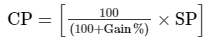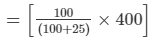= 40000/125

= Rs. 320

Thus, the actual cost of the article is Rs. 320.

PAGE NO 13.26:

Question 10:

A tradesman marks his goods at such a price that after allowing a discount of 15%, he makes a profit of 20%. What is the marked price of an article whose cost price is Rs 170?

Given,CP of the article  =  Rs. 170

Profit  =  20%

We know that,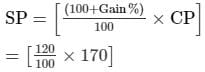= 20400/100

= Rs. 204

Let the MP of the article be Rs.  x .

Discount = 15%

Therefore, Discount = 15% of  x = 0.15 x

So, SP = MP−Discount

MP = SP + Discount

x = 204 + 0.15 x

x −0.15 x = 204

0.85 x = 204

x = Rs. 240

Thus, the marked price of the article is Rs. 240.

PAGE NO 13.26:

Question 11:

A shopkeeper marks his goods in such a way that after allowing a discount of 25% on the marked price, he still makes a profit of 50%. Find the ratio of the C.P. to the M.P.

Let C.P be Rs  x  and M.P be Rs y.

Gain%  =  50

We know that,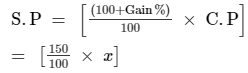=  3/2 x

Discount%  =  25

Discount  =  25% of y

=  Rs 0.25y

So, S.P  =  M.P − Discount

=  y − 0.25y

=  0.75y

So, S.P  =  0.75y

Also, S.P  =  3/2 x

Comparing both values for S.P., we get: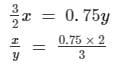= 1.5/3

= 1/2

Thus, C.P:M.P  =  1:2

PAGE NO 13.26:

Question 12:

A cycle dealer offers a discount of 10% and still makes a profit of 26%. What is the actual cost to him of a cycle whose marked price is Rs 840?

Given, MP of the cycle = Rs. 840

Discount = 10%

So, SP = MP ×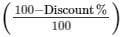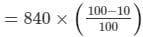= Rs. 756

Now, SP = Rs. 756 and Gain = 26%

So, CP =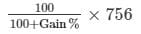= 100/126 × 756

= Rs. 600

Hence, the actual cost of the cycle is Rs. 600.

PAGE NO 13.26:

Question 13:

A shopkeeper allows 23% commision on his advertised price and still makes a profit of 10%. If he gains Rs 56 on one item, find his advertised price.

Let the CP of the item be Rs.  x .

Profit = 10%

SP = CP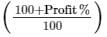SP = x (110/100)

SP = Rs.1.1 x

Again, Profit = SP−CP

Therefore, Profit = Rs. (1.1 x − x ) = Rs. 0.1 x

We get,0.1 x = 56 x

= Rs. 560

Now, the advertised price  = 1.1x /1−0.23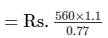= Rs. 800

Therefore, the advertised price of the item is Rs. 800.

PAGE NO 13.26:

Question 14:

A shopkeeper marks his goods at 40% above the cost price but allows a discount of 5% for cash payment to his customers. What actual profit does he make, if he receives Rs 1064 after paying the discount?

Let the original cost price of the item be Rs.  x .MP = x + 40 x 100 = 1.4 x

Discount = MP−SP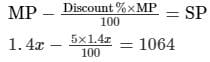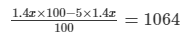So,  x (1.4)(0.95) = 1064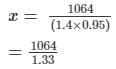= Rs. 800

Profit = Rs. (1064−800)

= Rs. 264

Thus, the actual profit by the shopkeeper is Rs. 264.

PAGE NO 13.26:

Question 15:

By selling a pair of earings at a discount of 25% on the marked price, a jeweller makes a profit of 16%. If the profit is Rs 48, what is the cost price? What is the marked price and the price at which the pair was eventually bought?

Let the cost price of the pair of earrings be Rs.  x .

Profit = 16%

Therefore, SP =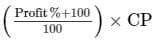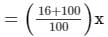= Rs. 116x/100

SP−CP = 48

⇒ 116x/100− x = 48

⇒ 16 x = 4800

⇒ x = Rs. 300

SP = 116x/100 = Rs. 348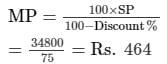= Rs. 464

Thus, CP of the pair of earrings = Rs.  x = Rs. 300

SP of the pair of earrings = Rs. 348

MP of the pair of earrings = Rs. 464

PAGE NO 13.26:

Question 16:

A publisher gives 32% discount on the printed price of a book to booksellers. What does a bookseller pay for a book whose printed price is Rs 275?

Discount allowed by the publisher = 32% on the printed price

Printed price = Rs. 275

So, 32% of 275

= 32/100 × 275

= Rs. 88

So, the bookseller pays  =  Rs. 275−Rs. 88

= Rs. 187 for a book

PAGE NO 13.26:

Question 17:

After allowing a discount of 20% on the marked price of a lamp, a trader loses 10%. By what percentage is the marked price above the cost price?

Let the CP of the lamp be Rs. 100.

Loss = 10% of CP = Rs. 10

So, SP = CP−Loss = Rs. 100−Rs. 10 = Rs. 90

The trader allows a discount of 20%. This means that when the MP is Rs. 100, the SP will be Rs. 80.

Now,If Rs. 80 is the SP, the MP = Rs. 100

If Re. 1 is the SP, the M.P = Rs. 100/80

If Rs. 90 is the SP, the MP = Rs. (100/80 × 90) = Rs. 112.50

Hence, the trader marks his goods at 12.5% above the cost price.

PAGE NO 13.26:

Question 18:

The list price of a table fan is Rs 480 and it is available to a retailer at 25% discount. For how much should a retailer sell it to gain 15%?

Marked price of the table fan = Rs. 480

Discount  = 25%

Therefore, cost price  =  25% of Rs. 480

25/100 × 480 = Rs. 360

It is given that the profit on the table fan is 15%.

Gain% = Gain/CP × 100

15 = Gain/360 × 100

Gain = Rs. 54

Gain = SP−CP

54 = SP−360

SP = Rs. 414

Thus, the retailer will sell the table fan for Rs. 414.

PAGE NO 13.27:

Question 19:

Rohit buys an item at 25% discount on the marked price. He sells it for Rs 660, making a profit of 10%. What is the marked price of the item?

Given,SP of the item  =  Rs. 660

Discount on the item  =  25%

Profit on the item  =  10%

We know,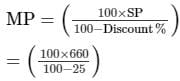= Rs. 880

Thus, the marked price of the item is Rs. 880.

PAGE NO 13.27:

Question 20:

A cycle merchant allows 20% discount on the marked price of the cycles and still makes a profit of 20%. If he gains Rs 360 over the sale of one cycle, find the marked price of the cycle.

Given,Gain on one cycle = Rs. 360

Gain = 20%

Gain% = Gain/CP × 100

20 = 360/CP × 100

CP = Rs. 1800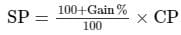SP = 120/100 × 1800 = Rs. 2160

SP = Rs. 2160

Discount = 20%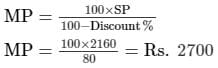Hence, the MP of one cycle is Rs. 2700.

PAGE NO 13.27:

Question 21:

Jyoti and Meena run a ready-made garment shop. They mark the garments at such a price that even after allowing a discount of 12.5%, they make a profit of 10%. Find the marked price of a suit which costs them Rs 1470.

Given,CP of the suit = Rs. 1470

Gain = 10%So, SP = Rs.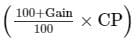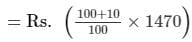= Rs. 1617

Now,SP = Rs. 1617

Discount = 12.5%

So, MP = Rs.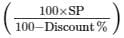= Rs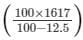= Rs. 1848

Therefore, the marked price of the suit is Rs. 1848.

PAGE NO 13.27:

Question 22:

What price should Aslam mark on a pair of shoes which costs him Rs 1200 so as to gain 12% after allowing a discount of 16%?

Given,CP of the pair of shoes = Rs. 1470

Gain = 12%

Discount = 16%

So, SP = Rs.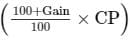= Rs.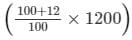= Rs. 1344

Now,SP of the pair of shoes = Rs. 1344

Discount = 16%

So, MP = Rs.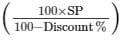= Rs.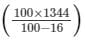= Rs. 1600

Aslam should sell the pair of shoes for Rs. 1600.

PAGE NO 13.27:

Question 23:

Jasmine allows 4% discount on the marked price of her goods and still earns a profit of 20%. What is the cost price of a shirt for her marked at Rs 850?

Given, MP of the shirt = Rs. 850

Discount = 4%

Discount allowed = Rs. (4100 × 850) = Rs. 34

Thus, SP of the shirt = Rs. (850−34) = Rs. 816

Now, Profit earned by Jasmine = 20%

Thus, CP =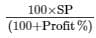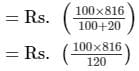= Rs. 680

Thus, the cost price of the shirt is Rs. 680.Given,

PAGE NO 13.27:

Question 24:

A shopkeeper offers 10% off-season discount to the customers and still makes a profit of 26%. What is the cost price for the shopkeeper on a pair of shoes marked at Rs 1120?

Given,MP of the pair of shoes = Rs. 1120

Discount  =  10%

So, SP = MP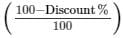= 1120 × 90/100

= Rs. 1008

Now,Profit = 26%

SP = Rs. 1008

Therefore, CP =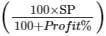Cost price  =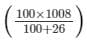= Rs. 800

The cost price of the pair of shoes will be Rs. 800.

PAGE NO 13.27:

Question 25:

A lady shopkeeper allows her customers 10% discount on the marked price of the goods and still gets a profit of 25%. What is the cost price of a fan for her marked at Rs 1250?

Given,MP of the fan = Rs. 1250

Discount = 10%

So, Discount  =  10% of 1250 = 0.10 × 1250

= Rs. 125

Since SP = MP−Discount,

SP = Rs. (1250−125)

= Rs. 1125

Now, SP of the fan  =  Rs. 1125

Profit  =  25%

CP =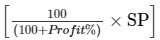= [100/125]1125

= Rs. 900

Thus, the cost price of the fan is Rs. 900.

The document RD Sharma Solutions - Chapter 13 - Proft, Loss, Discount and Value Added Tax (VAT) (Part -2), Class8 Notes | Study RD Sharma Solutions for Class 8 Mathematics - Class 8 is a part of the Class 8 Course RD Sharma Solutions for Class 8 Mathematics.
All you need of Class 8 at this link: Class 8Use Code STAYHOME200 and get INR 200 additional OFF

## RD Sharma Solutions for Class 8 Mathematics

88 docs

Track your progress, build streaks, highlight & save important lessons and more!

,

,

,

,

,

,

,

,

,

,

,

,

,

,

,

,

,

,

,

,

,

,

,

,

,

,

,

,

,

,

;